• 参数估计框图 粒子滤波思路
2021-12-24 19:22:31# 参数估计

## 非线性滤波

### 点估计

• 函数逼近，例如EKF
• 统计量逼近，例如UKF
• 随机模型逼近，例如CDF、DDF

### 概率密度估计

• 粒子滤波PF

• 直接对非线性函数对应的概率密度函数进行逼近

• 贝叶斯滤波

• 迭代关系式（3个）构成最优解，方程的解析解只有在某些特殊情况下才可以获得。f() h() 都为线性的，并且状态噪声是可叠加的、独立的、高斯的，这就是卡尔曼滤波，当噪声不服从高斯分布式，将无法得到滤波器最优的结论，最小方差的推导说明了无论噪声是何种概率密度分布，卡尔曼滤波器都是最优的线性滤波器。
• 蒙特卡洛方法（随机采样法、统计模拟法）

• 思想：将所求解的问题描述为某种概率统计模型化的随机变量，利用计算机进行模拟采样，从而获得问题的近似解。
• 重要性采样

• 从某一已知的、容易采样的函数中间接采样，这种函数称为重要性密度函数（IDF），这种采样方法称为重要性采样。
• 基本思想：通过一个相对简单分布函数的随机加权平均来近似计算目标分布函数的数学期望，该相对简单的分布函数被称为重要性密度函数或偏置函数。
• 目的：是一种受控的方式引入噪声偏置，增加稀有事件，减少运行时间。
• 重要性采样算法作为模特卡罗仿真中一种有效的快速仿真技术，主要作用在于降低给定仿真估计器的方差，从而减少仿真样本数，缩短仿真时间。
• 核心：偏置函数的选取，最优偏置函数可实现零方差，因此每个仿真实验能计算输出正确的期望值。
• 序贯重要性采样

• 克服重要性采样不能直接用来递推，主要因为估计的过程需要用到所有的量测信息。这种方法在k时刻采样时不会改动过去的状态序列。
• 粒子退化问题与重采样

• 粒子退化问题

• 经过若干次迭代后，除了少数几个粒子，大部分其他粒子的权值将小到可以忽略不计。
• 原因：重要性权值的方差将随时间的推移而增加。
• 选择合适的重要性密度函数

• 选择合适的重要性密度函数使方差最小化，从而使有效样本数最大化。
• 重采样

• 预先设定一个有效样本数的阈值，当有效粒子数低于阈值时进行重采样，其目的在于抑制权重较小的粒子，而关心权值较大的粒子。
• 总结

• 利用Monte Carlo方法，如果能从后验概率密度抽取N个样本，状态的PDF可以用经验分布逼近为 - 对样本集求均值。 但是通常不可能直接从状态的PDF采样，Bayes 重要性采样（IS）方法从一个容易采样的重要性分布函数独立抽取N个样本，逼近状态（带权值）的PDF，为了递推估计，选取重要性分布函数为先验(去掉没有积分)，从里面的p(xk/xk-1,yk)中抽取样本，称为序贯重要性采样（SIS），为了得到较好的估计，重要性分布应接近真实状态后验分布，因此重要性权的方差越小越好，针对重要性函数这样大的重要性分布，已经证明重要性权的方差随着时间增大，在极端情况下，经过若干次迭代后，某个权可能趋于1，其余权都趋于0，这称为权的退化现象，为了减轻权的退化，一个关键步骤是重要性分布函数的选择，在条件Xk-1和Yk下，使权的方差最小分布是q(xk/Xk-1,Yk)=p(xk/xk-1,yk)，称为最优重要性分布函数，最简单的重要性分布函数是状态的先验转移分布p(xk/xk-1)，称为Bootstrap滤波器。
• 由于SIS算法的退化不可避免，Gordon提出对样本重新采样，繁殖重要性权高的粒子，淘汰权低的粒子，从而抑制退化现象，最常用的重采样方法是采样-重要性重采样（SIR）方法，还有残差采样、最小方差采样等。 SIS和重采样就构成通常的SIR粒子滤波器，PF利用状态空间的一组带权值的随机样本（粒子）逼近状态变量的PDF，每个样本代表系统的一个可能状态，是基于Monte Carlo仿真的方法，具有较好的鲁棒性，适用于强非线性非高斯问题。
• 粒子滤波器的新变种

• 1、MCMC改进策略

• PF的重采样抑制了权的退化，但也引入了其它问题，重采样后，粒子不在独立（父代粒子一样），简单的收敛性结果不再成立，具有较高权的粒子被采样多次，粒子丧失多样性，极端情况下，经过若干次迭代后，所有粒子都坍塌到一个点上，这称为粒子的贫化。 重采样带来的粒子贫化问题使得表示状态PDF的粒子个数太少而不充分，无限增大粒子个数又不现实，Gordon等人提出对每个样本点增加高斯扰动，或着每次采样kN个样本，再从中重采样N个粒子，这些方法可以增加粒子的多样性，但是存在计算量过大甚至滤波器发散的问题。
• 子主题 2
• 2、Unscented粒子滤波器（UPF）

• 用UKF产生PF的重要性分布
• 3、辅助粒子滤波器（APF）

• 4、Rao-Blackwellised粒子滤波器（RBPF）

• 在维数很高的状态空间采样时，PF的效率很低，对某些状态空间模型，状态向量的一部分在其余部分的条件下的后验分布可以用解析方法求得，例如某些状态是条件线性 高斯模 型，可用 Kalman 滤波器得到条件后验分布 ，对另外部分状态用 PF，从而得到一种混合滤波器 ，降低了PF采样空间的维数，RBPF样本的重要性权的方差远远低于SIR方法的权的方差
• 5、正则化粒子滤波器（RPF）

• 通常的重采样是在离散分布中采样，Musso等提出了RPF，从连续分布中重采样，可以缓解粒子贫化 问题，在弱意义下收敛于最优滤波器。
• 6、高斯及高斯和粒子滤波器

• 高斯粒子滤波器用一个高斯分布逼近目标后验分布，如同EKF，高斯粒子滤波器也假定 目标分布是高斯分布，但不做线性化，通过粒子方法计算均值和方差，该方法比标准的PF容易执行，而性能又优于EKF．高斯和粒子滤波器使用一批高斯粒子滤波器通过加权高斯和分布逼近目标后验分布，结合了高斯混合滤波器及PF的优点 ．

## CKF

### 为了克服无迹滤波在高维系统中出现精度低的情况。算法 人工智能
更多相关内容
• 这是粒子滤波用于参数估计的代码，很适合初学者，里面集成了很多参数估计的思想
• 通过粒子滤波方法实时估计系统的状态值变化,结合最大似然方法计算静态参数的点估计,然后通过计算更新参数的似然值来动态更新步长序列。与在线EM参数估计算法(OEM)的实验结果比较,表明该算法具有更好的适应性和收敛...最大似然
• 本文将多径估计问题转化为状态空间模型下的参数估计问题,并利用粒子滤波(PF)进行多径估计。同时,为了克服标准PF存在粒子枯竭、导致估计结果可能收敛到错误值的问题,提出了基于差分进化改进粒子滤波(DEPF)的多径估计...
• 第 7章和第 8章为粒子滤波在目标跟踪、电池参数估计中的应用；第 章为粒子滤波在目标跟踪、电池参数估计中的应用；第 章为粒子滤波在目标跟踪、电池参数估计中的应用；第 章为粒子滤波在目标跟踪、电池参数估计中的...
• 针对包含多维微动参数的正弦调频项，提出改进的粒子滤波静态参数估计方法，通过设计自适应方差法和变化粒子数提升了算法效率，通过设计累积残差作为观测概率密度函数，实现了对非线性模型中多维参数的同时估计。...
• 粒子滤波进行参数估计，估计参数为一个，可以后期根据实际情况调整。
• PF）就是通过寻找一组在状态空间中传播的随机样本来近似的表示概率密度函数，用样本均值代替积分运算，进而获得系统状态的最小方差估计的过程，这些样本被称为“粒子”，故叫做粒子滤波粒子滤波(PF: Particle ...

# 1 简介

粒子滤波（Partical Filter，PF）就是通过寻找一组在状态空间中传播的随机样本来近似的表示概率密度函数，用样本均值代替积分运算，进而获得系统状态的最小方差估计的过程，这些样本被称为“粒子”，故叫做粒子滤波。

粒子滤波(PF: Particle Filter)的思想基蒙特卡洛方法(Monte Carlo methods)，它是利用粒子集来表示概率，可以用在任何形式的状态空间模型上。其核心思想是通过从后验概率中抽取的随机状态粒子来表达其分布，是一种顺序重要性采样法(Sequential Importance Sampling)。

# 2 基本算法

## 2.1 蒙特卡洛采样方法

假设我们能从一个目标概率分布中采样到一系列的样本（粒子），就能利用这些样本去估计这个分布的某些函数的期望值。例如：

蒙特卡洛的思想就是利用平均值代替积分：

在滤波、跟踪时，一般都是希望知道当前状态经过变换后的估计值

这里就是所谓的“粒子”。

## 2.2 重要性采样

上一节中给出了对于当前状态经过变换后的估计值的计算公式：

这里状态的分布可能未知，于是可以利用一个已知的可以采样的分布去采样，这里假设该参考采样为，于是有

其中，于是有

上面这个式子就可以利用蒙特卡洛方法去计算期望值。由蒙特卡洛方法代入到上面的式子得到

其中的表示归一化的权重值

上面的式子表示，对变量的变换之后的值，可以利用通过对一系列与该变量相关的粒子先进行变换，再通过加权求和的方式去估计，接下来的问题就是，如何求解权值

因为，而对的直接计算复杂，因此可以通过递推的方式求解：

上述公式中的是等价的。利用以上公式可以推出

这就是粒子的权值的递推公式。

## 2.3 序贯重要性采样

假设系统在时刻的状态只与时刻的状态有关，也就是具有马尔科夫性质，那么应有

于是粒子权值的递推公式就变成了以下形式

这样就能得出序贯重要性采样（Sequential Importance Sampling，SIS）的算法流程了：

1. 输入时刻的所有粒子的值及其相应的权重，以及时刻的观测值
2. 根据以下公式计算时刻所有的粒子的状态值以及相应的权重

## 2.4 重采样

在应用SIS滤波的过程中，存在一个粒子退化的问题，也就是说，经过几次迭代后，很多粒子的权重都变的很小，可以忽略掉，而只有少数的粒子的权重比较大。并且粒子权值的方差随着时间增大，状态空间中的有效粒子数较少，这样一来，无效采样粒子数的增加会使得大量的计算浪费在对后验滤波概率几乎不起作用的粒子上，最终使得算法的估计性能下降。

对于这样的粒子退化问题，通常采用有效粒子数来衡量粒子权值的退化程度，即

而上式又可以利用以下公式来近似计算

重采样的思路大致是这样的：舍去权重小的粒子，然后将权重大的粒子分割成若干个，最后使得分割得到的所有粒子的权重一致。基于未重采样的原粒子的后验概率密度为

经过重采样后变成

其中表示原粒子，表示该粒子应该分割的个数，表示重采样后的粒子，经过重采样后，所有粒子的权值都为。实现的基本思路是轮盘赌思想，举个简单的粒子：在时刻有3个粒子，权重分别为0.1，0.1，0.8，则它们的累计概率为，接着对上的均匀分布随机采样3个值，假设为0.15，0.38，0.54，那么，就应该让第二个粒子分割一次，第三个粒子分割两次。

利用上面的方法就能完成每一个时刻粒子的重采样。

# 3 粒子滤波算法

## 3.1 标准的粒子滤波算法

（1）粒子集的初始化，

对于，（表示粒子数，粒子数越大，效果越好，但也会使得计算量越大）由分布生成采样粒子

（2）对于，执行：

• 重要性采样：对于，从重要性概率密度函数生成采样粒子，然后计算粒子的权值并归一化得
• 重采样：对粒子集重采样得到新的粒子集，相应的权重为
• 估计输出：根据粒子集和相应的权重计算时刻的状态估计值，计算的公式为

## 3.2 SIR粒子滤波算法

SIR粒子滤波算法的流程如下：

（1）输入时刻的所有粒子的值及其相应的权重，以及时刻的观测值

（2）根据以下公式计算时刻所有粒子的状态值以及相应的权重

（3）计算粒子权重的和并归一化

（4）重采样

（5）根据以下公式估计状态

其中粒子的权重可以用以下的高斯分布计算

# 4 实际应用

现在我们假设在海上有一艘正在做匀速直线运动的船只，其相对于传感器的横纵坐标为为隐藏状态，无法直接获得，而传感器可以测量得到船只相对于传感器的距离和角度，传感器采样的时间间隔为，则：

状态向量，观测向量

状态转移方程和观测方程为：

这里给定距离传感器的噪声均值为，方差为；角度传感器的噪声均值为，方差为（单位弧度）；

粒子数量为，粒子散布范围为，采样时间点为个；

船只的初始状态为，四个状态量的噪声的方差分别为。仿真结果如下：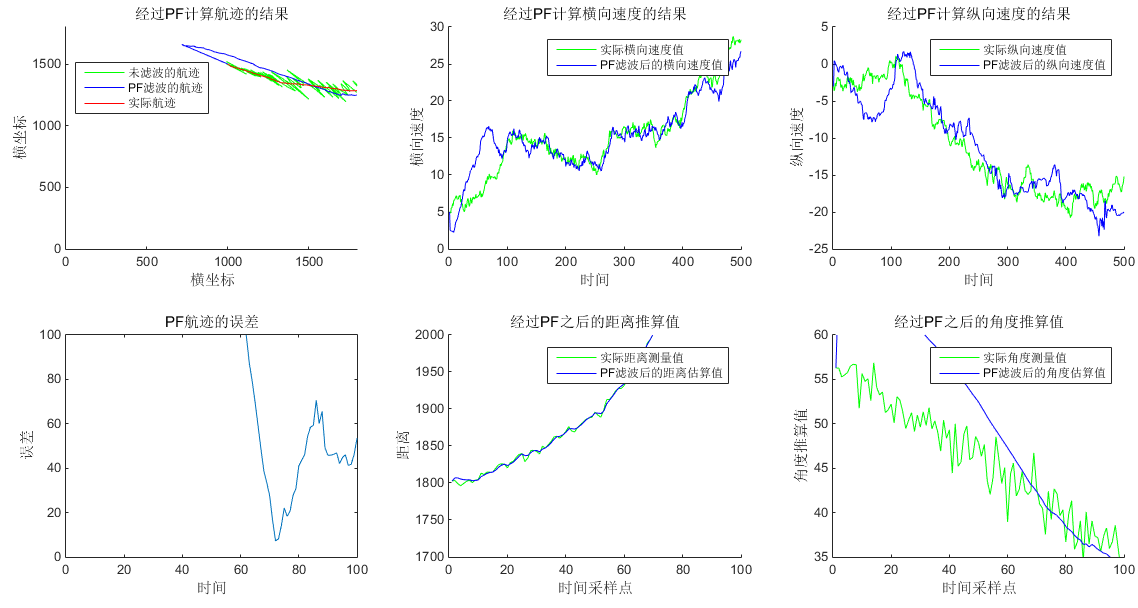从仿真结果可以看出，刚开始的一段数据的跟踪效果不好，这是因为初始的粒子是随机地在整个大范围中散布，这样就会有很多粒子给出不利于跟踪准确度的结果，但是随着时间的推移，粒子不断的调整和重采样，粒子滤波的跟踪结果最后开始向目标的真实位置收敛。

相比于EKF滤波，粒子滤波对速度量的跟踪更加准确。在这种情形下，总体来说滤波效果还是不错的，但是在实际应用中，对于船只运动的状态转移噪声的均值和协方差矩阵，以及传感器的观测噪声的均值和协方差矩阵，往往都是未知的，有很多情况都只有观测值而已，这样的情形下，就有必要利用观测值对噪声的统计量参数做出适当的估计（学习）。

# 5 参数估计（学习）

利用序贯的方式分别计算这八项乘积的累加和，再用于计算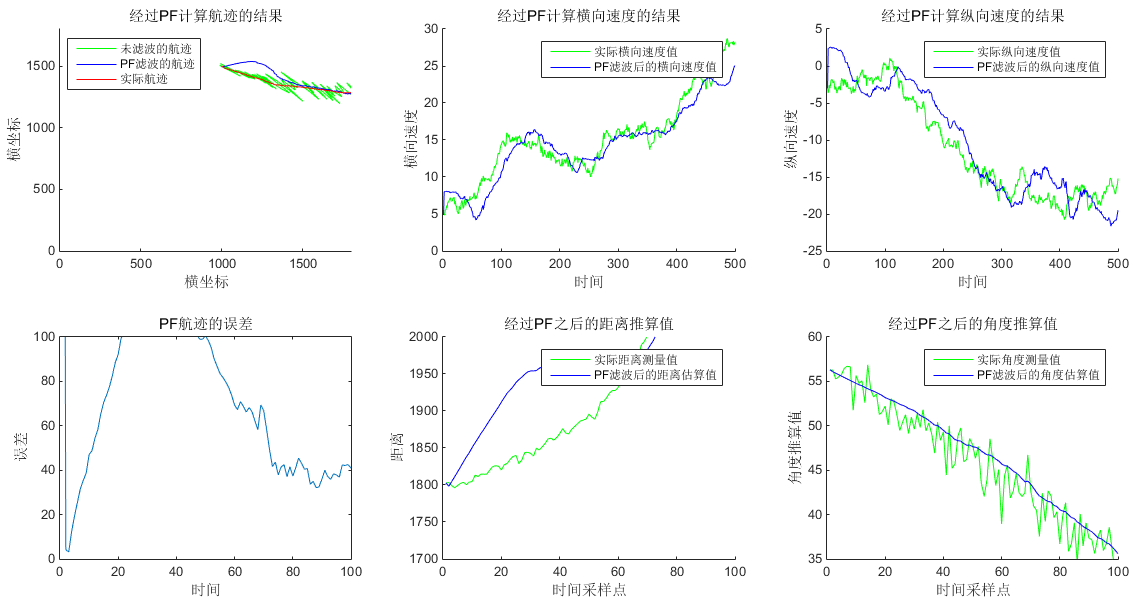# 6 总结

粒子滤波通过采用参考分布，随机产生大量粒子，近似状态的后验概率密度，从而得到系统的估计。粒子滤波并不需要计算Jacobi矩阵，也不用对系统做局部线性化，即可对状态进行估计，对复杂系统有较好的适用性。但是当对准确度要求很高的时候，粒子滤波所需要的粒子数量就很大，这会带来巨大的计算量，而且对于高维的状态，其计算量与维度呈现指数增长，为EKF的若干阶，而如果减少粒子数，又会使得滤波效果下降。于是就有了产生更优越更精确的粒子的无迹卡尔曼滤波UKF，将在下一篇博文中为读者解读。

# 7 参考文献

 Johansen, A.M., Doucet, A. & Davy, M. Stat Comput (2008) . Particle methods for maximum likelihood estimation in latent variable models.

原创性声明：本文属于作者原创性文章，小弟码字辛苦，转载还请注明出处。谢谢~

如果有哪些地方表述的不够得体和清晰，有存在的任何问题，亦或者程序存在任何考虑不周和漏洞，欢迎评论和指正，谢谢各路大佬。

需要代码和有需要相关技术支持的可咨询QQ：297461921

展开全文• 利用基于子空间分解的方法得到初始信道响应, 通过带反馈的粒子滤波方法更新信道响应和模型参数, 实现对OFDM信道的估计。仿真结果表明, 该方法不仅能对非时变信道进行有效估计, 而且可以较好地跟踪时变信道, 算法能够...正交频分复用
• 这会导致采样粒子贫化、粒子多样性缺失以及需要大量粒子才能进行比较准确的状态估计等问题,针对这些问题,提出了一种改进的蝶式算法优化粒子滤波算法。首先,将最新时刻观测信息引入蝴蝶香味公式中,以提高滤波精度;...
• 提出一种基于卡尔曼滤波与粒子滤波...通过计算复杂度分析及仿真实验验证,表明新方法与标准粒子滤波算法复杂度相当,但参数估计精度要高于标准粒子滤波以及扩展卡尔曼滤波算法,估计误差甚至要低于系统模型的克拉美劳下界.
• ## 改进粒子滤波算计及其matlab实现

千次阅读 多人点赞 2018-02-01 17:01:37
基本粒子滤波存在的问题 基本粒子滤波算法中普遍存在的问题是退化现象，这是因为粒子权值的方差会随着时间的迭代而不断增加。退化现象是不可避免的，经过若干次迭代，除了少数粒子外，其他粒子的权值可以忽略不计...

Github个人博客：https://joeyos.github.io

## 基本粒子滤波存在的问题

基本粒子滤波算法中普遍存在的问题是退化现象，这是因为粒子权值的方差会随着时间的迭代而不断增加。退化现象是不可避免的，经过若干次迭代，除了少数粒子外，其他粒子的权值可以忽略不计。退化的意味着如果继续跌代下去，那么大量的计算资源就会消耗在处理那些微不足道的粒子上。可以采取如下措施避免：

（1）增加粒子数
增加粒子数也叫增加采样点，粒子数目多，自然能全面反映粒子多样性，能延缓退化，但运算量增大。

（2）重采样的本质是增加粒子的多样性。SIR粒子滤波在这点上做得比SIS粒子滤波成功。引入重采样机制，基本上避免了粒子丧失多样性的可能。重采样技术和选择合理的建议密度是同时采用的。

（3）选择合理的建议密度
基本粒子滤波的前提假设：重要性重采样能够从一个合理的后验建议密度分布中采样得到一组样本点集合，而且这组样本点集合能够很好地覆盖真实状态。如果这些假设条件不能满足，粒子滤波算法的效果就要下降；因此，如果能找到一个最优的建议密度分布函数，引导重采样做正确的采样分布，那么就能保证样本集合的有效性，也就保证了滤波的最终质量。

## 建议密度函数

介绍两种从建议密度函数的角度来改进粒子滤波算法的方法，分别用扩展卡尔曼EKF和无迹卡尔曼UKF来做建议密度函数，从而改进算法性能。

## EPF算法

扩展卡尔曼是一种局部线性化的方法，它通过一阶泰勒展开式实现，它是一种递归的最小均方误差MMSE估计方法，要求系统是近似高斯后验分布模型。在粒子滤波算法框架下，EKF算法主要用于为每个粒子产生符合建议密度分布，称为扩展卡尔曼粒子滤波（EPF）。初始化-重要性采样-重采样-输出。

## UPF算法

无迹卡尔曼滤波也是一种递归的最小均方误差估计，利用UKF来改进粒子滤波算法称为无迹卡尔曼粒子滤波（UPF）。初始化-重要性采样-重采样-输出。

UPF算法的误差较低，但计算时间较长。

## 算法实现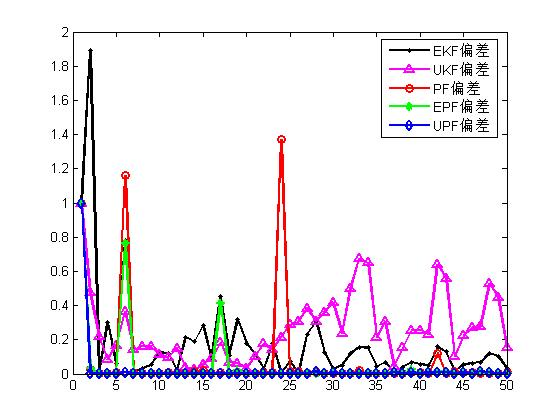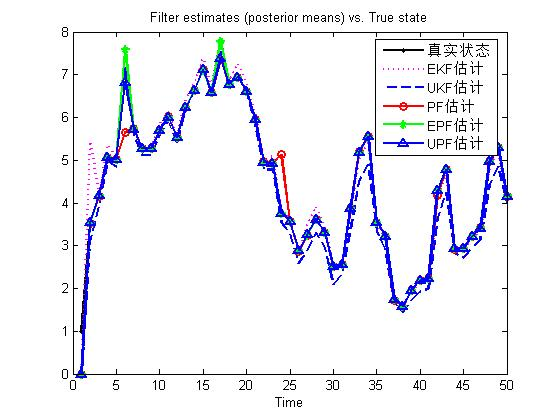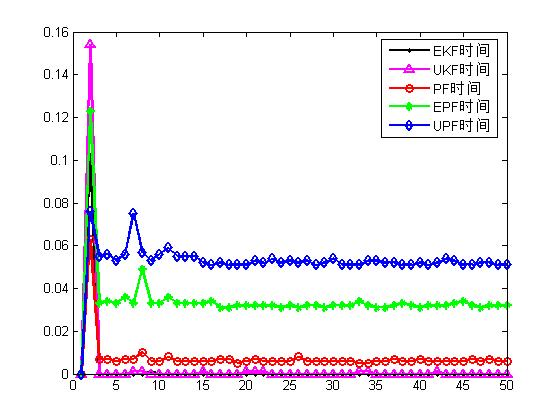1.main.m

%%%%%%%%%%%%%%%%%%%%%%%%%%%%%%%%%%%%%%%%%%%%%%%%%%%%%%%%%%%%%%%%%%%%%%%%%
%% 主函数
%%%%%%%%%%%%%%%%%%%%%%%%%%%%%%%%%%%%%%%%%%%%%%%%%%%%%%%%%%%%%%%%%%%%%%%%%%
% 功能说明：ekf,ukf,pf,epf,upf算法的综合比较程序
function main

rand('seed',3);
randn('seed',6);
%error('下面的参数T请参考书中的值设置，然后删除本行代码')
T = 50;
R =  1e-5;

g1 = 3;
g2 = 2;

X = zeros(1,T);
Z = zeros(1,T);
processNoise = zeros(T,1);
measureNoise = zeros(T,1);
X(1) = 1;
P0 = 3/4;

Qekf=10*3/4;
Rekf=1e-1;
Xekf=zeros(1,T);
Pekf = P0*ones(1,T);
Tekf=zeros(1,T);

Qukf=2*3/4;
Rukf=1e-1;
Xukf=zeros(1,T);
Pukf = P0*ones(1,T);
Tukf=zeros(1,T);

N=200;
Xpf=zeros(1,T);
Xpfset=ones(T,N);
Tpf=zeros(1,T);

Xepf=zeros(1,T);
Xepfset=ones(T,N);
Pepf = P0*ones(T,N);
Tepf=zeros(1,T);

Xupf=zeros(1,T);
Xupfset=ones(T,N);
Pupf = P0*ones(T,N);
Tupf=zeros(1,T);

for t=2:T
processNoise(t) =  gengamma(g1,g2);
measureNoise(t) =  sqrt(R)*randn;

X(t) = feval('ffun',X(t-1),t) +processNoise(t);

Z(t) = feval('hfun',X(t),t) + measureNoise(t);

tic
[Xekf(t),Pekf(t)]=ekf(Xekf(t-1),Z(t),Pekf(t-1),t,Qekf,Rekf);
Tekf(t)=toc;

tic
[Xukf(t),Pukf(t)]=ukf(Xukf(t-1),Z(t),Pukf(t-1),Qukf,Rukf,t);
Tukf(t)=toc;

tic
[Xpf(t),Xpfset(t,:)]=pf(Xpfset(t-1,:),Z(t),N,t,R,g1,g2);
Tpf(t)=toc;

tic
[Xepf(t),Xepfset(t,:),Pepf(t,:)]=epf(Xepfset(t-1,:),Z(t),t,Pepf(t-1,:),N,R,Qekf,Rekf,g1,g2);
Tepf(t)=toc;

tic
[Xupf(t),Xupfset(t,:),Pupf(t,:)]=upf(Xupfset(t-1,:),Z(t),t,Pupf(t-1,:),N,R,Qukf,Rukf,g1,g2);
Tupf(t)=toc;
end;

ErrorEkf=abs(Xekf-X);
ErrorUkf=abs(Xukf-X);
ErrorPf=abs(Xpf-X);
ErrorEpf=abs(Xepf-X);
ErrorUpf=abs(Xupf-X);

figure
hold on;box on;
p1=plot(1:T,X,'-k.','lineWidth',2);
p2=plot(1:T,Xekf,'m:','lineWidth',2);
p3=plot(1:T,Xukf,'--','lineWidth',2);
p4=plot(1:T,Xpf,'-ro','lineWidth',2);
p5=plot(1:T,Xepf,'-g*','lineWidth',2);
p6=plot(1:T,Xupf,'-b^','lineWidth',2);
legend([p1,p2,p3,p4,p5,p6],'真实状态','EKF估计','UKF估计','PF估计','EPF估计','UPF估计')
xlabel('Time','fontsize',10)
title('Filter estimates (posterior means) vs. True state','fontsize',10)

figure
hold on;box on;
p1=plot(1:T,ErrorEkf,'-k.','lineWidth',2);
p2=plot(1:T,ErrorUkf,'-m^','lineWidth',2);
p3=plot(1:T,ErrorPf,'-ro','lineWidth',2);
p4=plot(1:T,ErrorEpf,'-g*','lineWidth',2);
p5=plot(1:T,ErrorUpf,'-bd','lineWidth',2);
legend([p1,p2,p3,p4,p5],'EKF偏差','UKF偏差','PF偏差','EPF偏差','UPF偏差')

figure
hold on;box on;
p1=plot(1:T,Tekf,'-k.','lineWidth',2);
p2=plot(1:T,Tukf,'-m^','lineWidth',2);
p3=plot(1:T,Tpf,'-ro','lineWidth',2);
p4=plot(1:T,Tepf,'-g*','lineWidth',2);
p5=plot(1:T,Tupf,'-bd','lineWidth',2);
legend([p1,p2,p3,p4,p5],'EKF时间','UKF时间','PF时间','EPF时间','UPF时间')
%%%%%%%%%%%%%%%%%%%%%%%%%%%%%%%%%%%%%%%%%%%%%%%%%%%%%%%%%%%%%%%%%%%%%%%%%%


2.ekf.m

function [Xekf,Pout]=ekf(Xin,Z,Pin,t,Qekf,Rekf)

Xpre=feval('ffun',Xin,t);
Jx=0.5;

Pekfpre = Qekf + Jx*Pin*Jx';

Zekfpre= feval('hfun',Xpre,t);

if t<=30
Jy = 2*0.2*Xpre;
else
Jy = 0.5;
end

M = Rekf + Jy*Pekfpre*Jy';

K = Pekfpre*Jy'*inv(M);

Xekf=Xpre+K*(Z-Zekfpre);

Pout = Pekfpre - K*Jy*Pekfpre;
%%%%%%%%%%%%%%%%%%%%%%%%%%%%%%%%%%%%%%%%%%%%%%%%%%%%%%%%%%%%%%%%%%%%%%%%%%


3.ukf.m

% 无迹卡尔曼滤波算法
function [Xout,Pout]=ukf(Xin,Z,Pin,Qukf,Rukf,t)
alpha = 1;
beta  = 0;
kappa = 2;

states       = size(Xin(:),1);
observations = size(Z(:),1);
vNoise       = size(Qukf,2);
wNoise       = size(Rukf,2);
noises       = vNoise+wNoise;

if (noises)
N=[Qukf zeros(vNoise,wNoise); zeros(wNoise,vNoise) Rukf];
PQ=[Pin zeros(states,noises);zeros(noises,states) N];
xQ=[Xin;zeros(noises,1)];
else
PQ=Pin;
xQ=Xin;
end;

[xSigmaPts, wSigmaPts, nsp] = scaledSymmetricSigmaPoints(xQ, PQ, alpha, beta, kappa);

wSigmaPts_xmat = repmat(wSigmaPts(:,2:nsp),states,1);
wSigmaPts_zmat = repmat(wSigmaPts(:,2:nsp),observations,1);

xPredSigmaPts = feval('ffun',xSigmaPts(1:states,:),t)+xSigmaPts(states+1:states+vNoise,:);
zPredSigmaPts = feval('hfun',xPredSigmaPts,t)+xSigmaPts(states+vNoise+1:states+noises,:);

xPred = sum(wSigmaPts_xmat .* (xPredSigmaPts(:,2:nsp) - repmat(xPredSigmaPts(:,1),1,nsp-1)),2);
zPred = sum(wSigmaPts_zmat .* (zPredSigmaPts(:,2:nsp) - repmat(zPredSigmaPts(:,1),1,nsp-1)),2);
xPred=xPred+xPredSigmaPts(:,1);
zPred=zPred+zPredSigmaPts(:,1);

exSigmaPt = xPredSigmaPts(:,1)-xPred;
ezSigmaPt = zPredSigmaPts(:,1)-zPred;

PPred   = wSigmaPts(nsp+1)*exSigmaPt*exSigmaPt';
PxzPred = wSigmaPts(nsp+1)*exSigmaPt*ezSigmaPt';
S       = wSigmaPts(nsp+1)*ezSigmaPt*ezSigmaPt';

exSigmaPt = xPredSigmaPts(:,2:nsp) - repmat(xPred,1,nsp-1);
ezSigmaPt = zPredSigmaPts(:,2:nsp) - repmat(zPred,1,nsp-1);
PPred     = PPred + (wSigmaPts_xmat .* exSigmaPt) * exSigmaPt';
S         = S + (wSigmaPts_zmat .* ezSigmaPt) * ezSigmaPt';
PxzPred   = PxzPred + exSigmaPt * (wSigmaPts_zmat .* ezSigmaPt)';

K  = PxzPred / S;

inovation = Z - zPred;

Xout = xPred + K*inovation;

Pout = PPred - K*S*K';
%%%%%%%%%%%%%%%%%%%%%%%%%%%%%%%%%%%%%%%%%%%%%%%%%%%%%%%%%%%%%%%%%%%%%%%%%%


4.pf.m

% 基本粒子滤波算法
function [Xo,Xoset]=pf(Xiset,Z,N,k,R,g1,g2)

tic

resamplingScheme=1;

Zpre=ones(1,N);
Xsetpre=ones(1,N);
w = ones(1,N);

for i=1:N
Xsetpre(i) = feval('ffun',Xiset(i),k) + gengamma(g1,g2);
end;

for i=1:N,
Zpre(i) = feval('hfun',Xsetpre(i),k);
w(i) = inv(sqrt(R)) * exp(-0.5*inv(R)*((Z-Zpre(i))^(2))) ...
+ 1e-99;
end;
w = w./sum(w);

if resamplingScheme == 1
outIndex = residualR(1:N,w');
elseif resamplingScheme == 2
outIndex = systematicR(1:N,w');
else
outIndex = multinomialR(1:N,w');
end;

Xoset = Xsetpre(outIndex);
Xo=mean(Xoset);
%%%%%%%%%%%%%%%%%%%%%%%%%%%%%%%%%%%%%%%%%%%%%%%%%%%%%%%%%%%%%%%%%%%%%%%%%%


5.epf.m

% 用EKF改进的粒子滤波算法--EPF
% 用EKF产生建议分布
% 输入参数说明：
%    Xiset是上t-1时刻的粒子集合，Z是t时刻的观测
%    Pin对应Xiset粒子集合的方差\
% 输出参数说明：
%    Xo是epf算法最终的估计结果
%    Xoset是k时刻的粒子集合，其均值就是Xo
%    Pout是Xoset对应的方差
function [Xo,Xoset,Pout]=epf(Xiset,Z,t,Pin,N,R,Qekf,Rekf,g1,g2)

resamplingScheme=1;

Zpre=ones(1,N);
Xsetpre=ones(1,N);
w = ones(1,N);

Pout=ones(1,N);
Xekf=ones(1,N);
Xekf_pre=ones(1,N);

for i=1:N

[Xekf(i),Pout(i)]=ekf(Xiset(i),Z,Pin(i),t,Qekf,Rekf);

Xsetpre(i)=Xekf(i)+sqrtm(Pout(i))*randn;
end

for i=1:N,

Zpre(i) = feval('hfun',Xsetpre(i),t);

lik = inv(sqrt(R)) * exp(-0.5*inv(R)*((Z-Zpre(i))^(2)))+1e-99;
prior = ((Xsetpre(i)-Xiset(i))^(g1-1)) * exp(-g2*(Xsetpre(i)-Xiset(i)));
proposal = inv(sqrt(Pout(i))) * ...
exp(-0.5*inv(Pout(i)) *((Xsetpre(i)-Xekf(i))^(2)));
w(i) = lik*prior/proposal;
end;

w= w./sum(w);

if resamplingScheme == 1
outIndex = residualR(1:N,w');
elseif resamplingScheme == 2
outIndex = systematicR(1:N,w');
else
outIndex = multinomialR(1:N,w');
end;

Xoset = Xsetpre(outIndex);
Pout = Pout(outIndex);

Xo = mean(Xoset);
%%%%%%%%%%%%%%%%%%%%%%%%%%%%%%%%%%%%%%%%%%%%%%%%%%%%%%%%%%%%%%%%%%%%%%%%%%


6.upf.m

% 用UKF改进的粒子滤波算法--UPF
% 用UKF产生建议分布
% 输入参数说明：
%    Xiset是上t-1时刻的粒子集合，Z是t时刻的观测
%    Pin对应Xiset粒子集合的方差\
% 输出参数说明：
%    Xo是upf算法最终的估计结果
%    Xoset是k时刻的粒子集合，其均值就是Xo
%    Pout是Xoset对应的方差
function [Xo,Xoset,Pout]=upf(Xiset,Z,t,Pin,N,R,Qukf,Rukf,g1,g2)

resamplingScheme=1;

Xukf=ones(1,N);
Xset_pre=ones(1,N);
Zpre=ones(1,N);
for i=1:N

[Xukf(i),Pout(i)]=ukf(Xiset(i),Z,Pin(i),Qukf,Rukf,t);

Xset_pre(i) = Xukf(i) + sqrtm(Pout(i))*randn;
end

for i=1:N

Zpre(i) = feval('hfun',Xset_pre(i),t);

lik = inv(sqrt(R)) * exp(-0.5*inv(R)*((Z-Zpre(i))^(2)))+1e-99;
prior = ((Xset_pre(i)-Xiset(i))^(g1-1)) * exp(-g2*(Xset_pre(i)-Xiset(i)));
proposal = inv(sqrt(Pout(i))) * ...
exp(-0.5*inv(Pout(i)) *((Xset_pre(i)-Xukf(i))^(2)));
w(i) = lik*prior/proposal;
end;

w = w./sum(w);

if resamplingScheme == 1
outIndex = residualR(1:N,w');
elseif resamplingScheme == 2
outIndex = systematicR(1:N,w');
else
outIndex = multinomialR(1:N,w');
end;

Xoset = Xset_pre(outIndex);
Pout = Pout(outIndex);

Xo = mean(Xoset);
%%%%%%%%%%%%%%%%%%%%%%%%%%%%%%%%%%%%%%%%%%%%%%%%%%%%%%%%%%%%%%%%%%%%%%%%%%



7.ffun.m

%%%%%%%%%%%%%%%%%%%%%%%%%%%%%%%%%%%%%%%%%%%%%%%%%%%%%%%%%%%%%%%%%%%%%%%%%%
% 状态方程函数
function [y] = ffun(x,t);

if nargin < 2
error('Not enough input arguments.');
end
beta = 0.5;
y = 1 + sin(4e-2*pi*t) + beta*x;


8.hfun.m

%%%%%%%%%%%%%%%%%%%%%%%%%%%%%%%%%%%%%%%%%%%%%%%%%%%%%%%%%%%%%%%%%%%%%%%%%%
% 观测方程函数
function [y] = hfun(x,t);

if nargin < 2,
error('Not enough input arguments.');
end
if t<=30
y = (x.^(2))/5;
else
y = -2 + x/2;
end;


9.gengamma.m

%%%%%%%%%%%%%%%%%%%%%%%%%%%%%%%%%%%%%%%%%%%%%%%%%%%%%%%%%%%%%%%%%%%%%%%%%%
% 产生一个符合gamma分布的噪声
function x = gengamma(alpha, beta)

if (alpha==1)
x = -log(1-rand(1,1))/beta;
return
end
flag=0;
if (alpha<1)
flag=1;
alpha=alpha+1;
end
gamma=alpha-1;
eta=sqrt(2.0*alpha-1.0);
c=.5-atan(gamma/eta)/pi;
aux=-.5;
while(aux<0)
y=-.5;
while(y<=0)
u=rand(1,1);
y = gamma + eta * tan(pi*(u-c)+c-.5);
end
v=-log(rand(1,1));
aux=v+log(1.0+((y-gamma)/eta)^2)+gamma*log(y/gamma)-y+gamma;
end;

if (flag==1)
x = y/beta*(rand(1))^(1.0/(alpha-1));
else
x = y/beta;
end


10.scaledSymmetricSigmaPoints.m

function [xPts, wPts, nPts] = scaledSymmetricSigmaPoints(x,P,alpha,beta,kappa)
n    = size(x(:),1);
nPts = 2*n+1;
kappa = alpha^2*(n+kappa)-n;
wPts=zeros(1,nPts);
xPts=zeros(n,nPts);
Psqrtm=(chol((n+kappa)*P))';
xPts=[zeros(size(P,1),1) -Psqrtm Psqrtm];
xPts = xPts + repmat(x,1,nPts);
wPts=[kappa 0.5*ones(1,nPts-1) 0]/(n+kappa);
wPts(nPts+1) = wPts(1) + (1-alpha^2) + beta;
%%%%%%%%%%%%%%%%%%%%%%%%%%%%%%%%%%%%%%%%%%%%%%%%%%%%%%%%%%%%%%%%%%%%%%%%%%


11.multinomialR.m

%% 多项式重采样
function outIndex = multinomialR(inIndex,q);

error('下面的参数nargin请参考书中的值设置，然后删除本行代码')
if nargin < 0, error('Not enough input arguments.'); end

[S,arb] = size(q);

N_babies= zeros(1,S);
cumDist= cumsum(q');

u = fliplr(cumprod(rand(1,S).^(1./(S:-1:1))));
j=1;
for i=1:S
while (u(1,i)>cumDist(1,j))
j=j+1;
end
N_babies(1,j)=N_babies(1,j)+1;
end;

index=1;
for i=1:S
if (N_babies(1,i)>0)
for j=index:index+N_babies(1,i)-1
outIndex(j) = inIndex(i);
end;
end;
index= index+N_babies(1,i);
end


12.residualR.m

%% 随机重采样
function outIndex = residualR(inIndex,q);
if nargin < 2, error('Not enough input arguments.'); end

[S,arb] = size(q);

N_babies= zeros(1,S);

q_res = S.*q';
N_babies = fix(q_res);

N_res=S-sum(N_babies);
if (N_res~=0)
q_res=(q_res-N_babies)/N_res;
cumDist= cumsum(q_res);

u = fliplr(cumprod(rand(1,N_res).^(1./(N_res:-1:1))));
j=1;
for i=1:N_res
while (u(1,i)>cumDist(1,j))
j=j+1;
end
N_babies(1,j)=N_babies(1,j)+1;
end;
end;

index=1;
for i=1:S
if (N_babies(1,i)>0)
for j=index:index+N_babies(1,i)-1
outIndex(j) = inIndex(i);
end;
end;
index= index+N_babies(1,i);
end


13.systematicR.m

%% 系统重采样
function outIndex = systematicR(inIndex,wn);

error('下面的参数nargin 请参考书中的值设置，然后删除本行代码')
if nargin < 0, error('Not enough input arguments.'); end

wn=wn';
[arb,N] = size(wn);

N_children=zeros(1,N);
label=zeros(1,N);
label=1:1:N;

s=1/N;
auxw=0;
auxl=0;
li=0;
T=s*rand(1);
j=1;
Q=0;
i=0;

u=rand(1,N);
while (T<1)
if (Q>T)
T=T+s;
N_children(1,li)=N_children(1,li)+1;
else

i=fix((N-j+1)*u(1,j))+j;

auxw=wn(1,i);
li=label(1,i);

Q=Q+auxw;

wn(1,i)=wn(1,j);
label(1,i)=label(1,j);

j=j+1;
end
end

index=1;
for i=1:N
if (N_children(1,i)>0)
for j=index:index+N_children(1,i)-1
outIndex(j) = inIndex(i);
end;
end;
index= index+N_children(1,i);
end


此博客均属原创或译文，欢迎转载但请注明出处
Github个人博客：https://joeyos.github.io

展开全文目标跟踪与检测
• 提出了一种基于粒子滤波的多径时变信道盲均衡算法,并在此基础上进行扩展,提出了一种基于延迟抽样的盲均衡算法。新算法的贡献可总结为:推导出对称α稳定分布(SαS)噪声下对传输码元进行最大后验估计的盲贯序算法;对S...
• 点击上方“计算机视觉工坊”，选择“星标”干货第一时间送达一、前言粒子滤波（particle filter）是一种常见的滤波算法，广泛应用于目标跟踪、移动机器人等领域。网络上有不少关于粒子滤...

点击上方“计算机视觉工坊”，选择“星标”

干货第一时间送达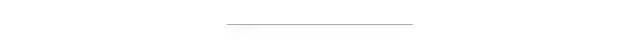# 一、前言

粒子滤波（particle filter）是一种常见的滤波算法，广泛应用于目标跟踪、移动机器人等领域。网络上有不少关于粒子滤波的资料，但大多是直接给出了粒子滤波的相关公式和证明，或较为直观上的解释。作者在学习粒子滤波的过程中对一些概念和操作时常感到突兀，后来发现想要完整了解粒子滤波，需要首先了解前因，逐渐深入才能理解粒子滤波，而不是直接学习粒子滤波这个方法。

本文将侧重从“粒子滤波是怎么来的”这个问题介绍粒子滤波。限于篇幅与易懂性，对一些概念并没有展开介绍，读者在了解基本思路后可以根据给出的资料深入学习。本文包含了作者自己不严谨的理解与阐述，如有疏漏，望批评指正。

# 二、对“滤波”的一些介绍

## 2.1 何为“滤波”？

贝叶斯滤波、卡尔曼滤波、粒子滤波……种种这些滤波方法，都涉及到了“滤波”这个词。那么到底什么是滤波，不同的领域有不同的定义。比如在信号系统领域，滤波是指将信号中特定波段的频率滤除的操作。而在移动机器人领域，我暂时没有看到较为严格的定义。我认为可以姑且理解为：通过不断地观测，使得对目标状态的估计变得更加准确。

## 2.2 贝叶斯滤波

卡尔曼滤波与粒子滤波都是基于贝叶斯滤波框架下的滤波算法。讲粒子滤波便不得不提贝叶斯滤波。贝叶斯滤波的基本思想是根据上一时刻的状态对当前状态进行预测，并根据此时的观测进行更新。基本算法是：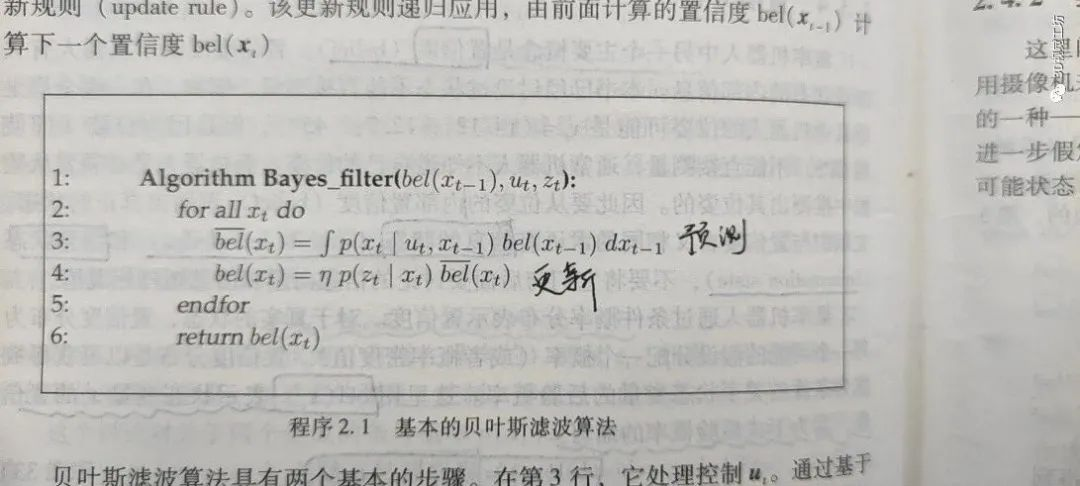（图片来源：《概率机器人》）

可以看出，在预测部分需要求一个积分，而这个积分往往很难求。所以显有方法可以直接利用原始的贝叶斯进行处理。

## 2.3 卡尔曼滤波

卡尔曼滤波也是非常庞大的一块内容，这里不展开介绍。只在这里说明，卡尔曼滤波是贝叶斯滤波在线性高斯系统下的一种滤波算法。而对于非线性系统，则衍生出来了扩展卡尔曼滤波。同时指出，无论是卡尔曼还是扩展卡尔曼滤波，都是参数化的滤波方法，对于无法用参数化进行表示的，则采用粒子滤波。粒子滤波是一种无参的滤波算法。

# 三、积分计算：从蒙特卡洛说起

## 3.1 分段近似法求积分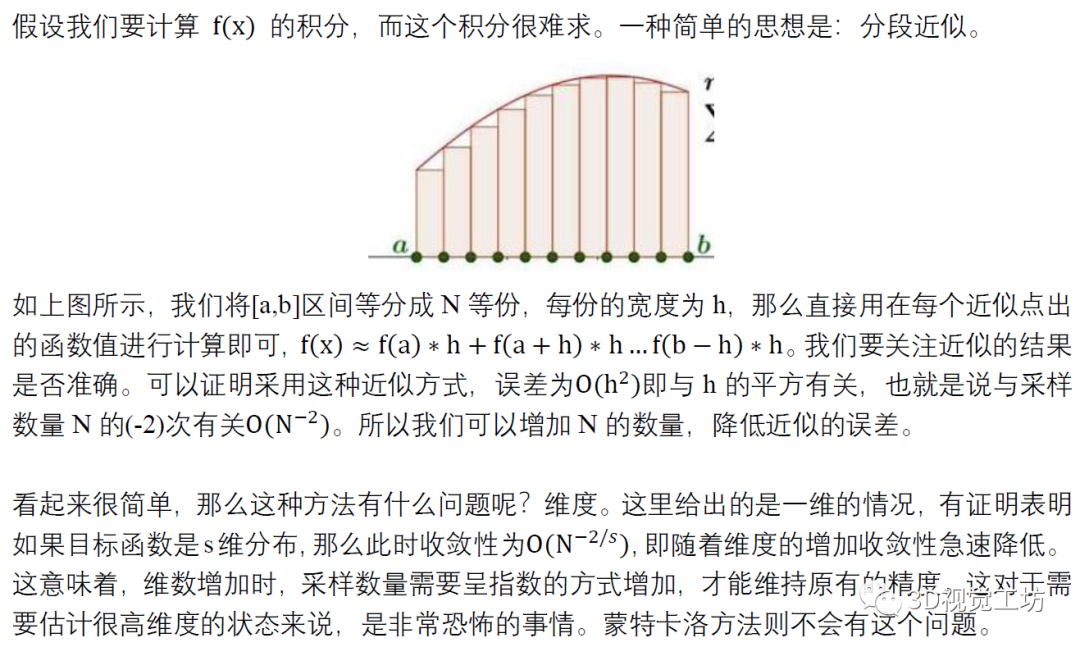## 3.2 蒙特卡洛采样求积分

（此处略过蒙特卡洛基本原理）

### 3.2.1 简单的均匀采样

求积分和求期望是相同的。假设我们对一个分布求取积分，采用最简单的采样方式——均匀采样。我们求取在x满足均匀分布u(x)时，f(x)在[a,b]的期望I。按照分布u(x)进行N次随机采样：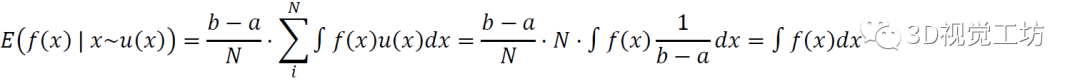可以发现最后一项对f(x)的积分，就是x的期望。所以我们可以发现，当我们按照均匀分布u(x)对x进行大量采样，计算对应的f(x)的平均值，就是f(x)的积分。

### 3.2.2 任意分布的采样

下面我们研究，如果不是按照均匀分布u(x)采样，而是任意分布p(x)进行采样，结果如何。此时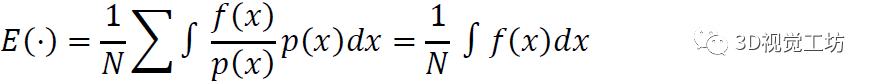依旧与原始的积分相同。所以我们得出了重要的结论：在蒙特卡洛时，我们可以按照任意分布进行采样，再计算对应f(x)的积分。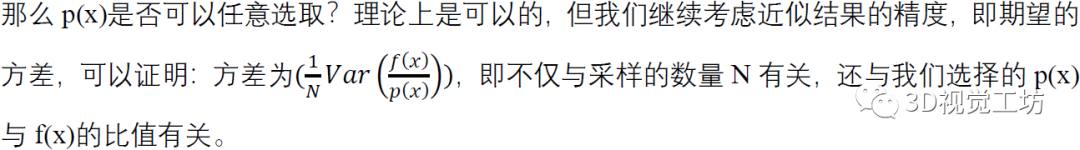这一点很好理解，如果我们选择的分布p(x)就是真实的分布，那么我们从p(x)进行采样，就和直接从真实分布进行采样是一样的，积分结果当然是没有误差的。这提醒我们，在选取p(x)分布时要尽可能的与实际分布接近，从而极大程度的降低方差，从而减少需要采样的数量。

# 四、重要性采样与序列重要性采样

## 4.1 重要性采样(Importance Sampling, IS)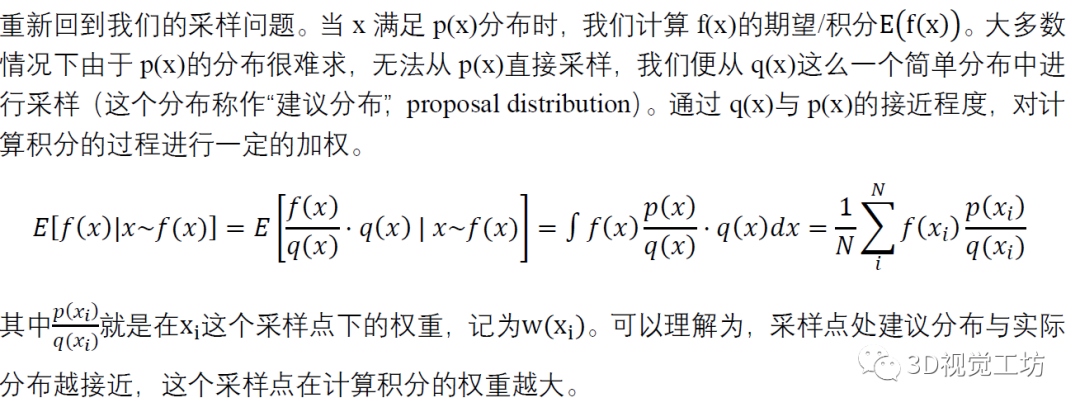## 4.2 序列重要性采样(Sequential Importance Sampling, SIS)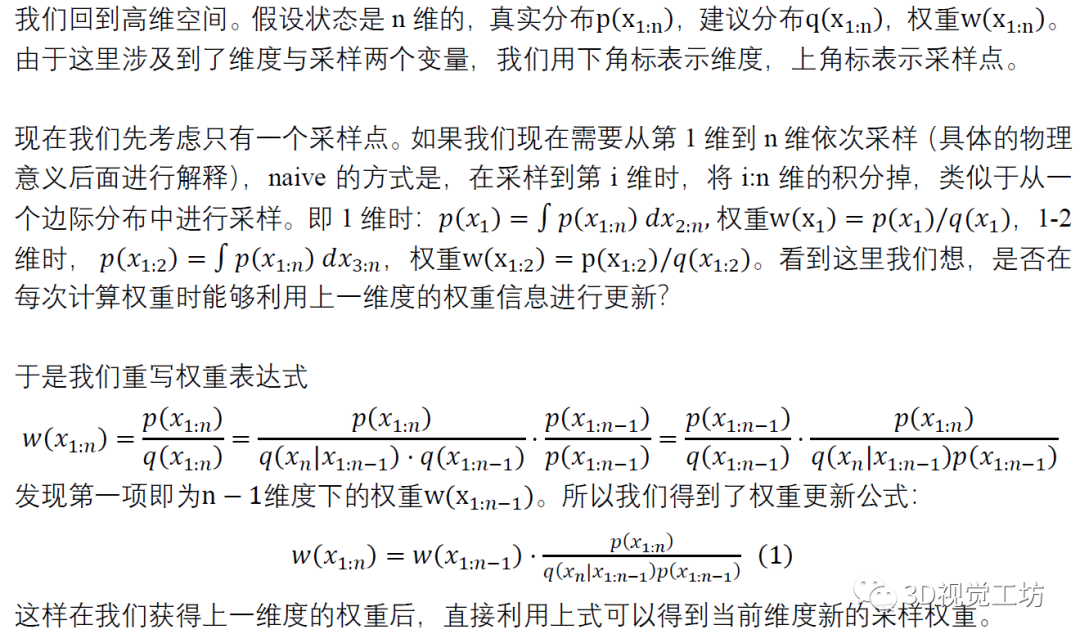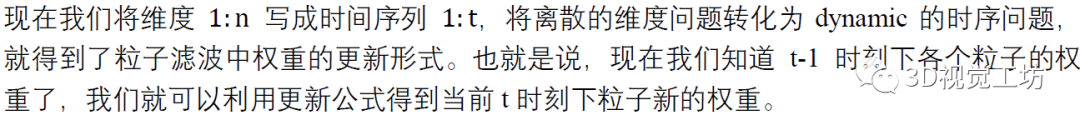## 4.3 重采样(Resampling)

在实际过程中，我们发现利用权重更新公式进行更新时，在几次迭代之后，权重的分布会极其不均匀，出现个别粒子权重很大接近于1，而其他的都接近于0的情况。这时候采用了一种“重采样”策略，即每次权重更新之后，根据当前权重对所有粒子进行重采样，之后将所有权重设定为相同。这样我们用粒子的数量代替了粒子的权重，避免了权重的不均匀。

# 5. 粒子滤波(Particle Filter)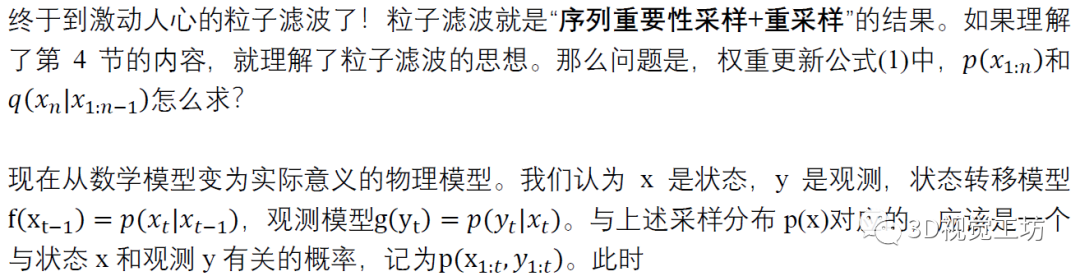此时对权重更新公式进行变形（在不产生歧义情况下部分内容用点省略）：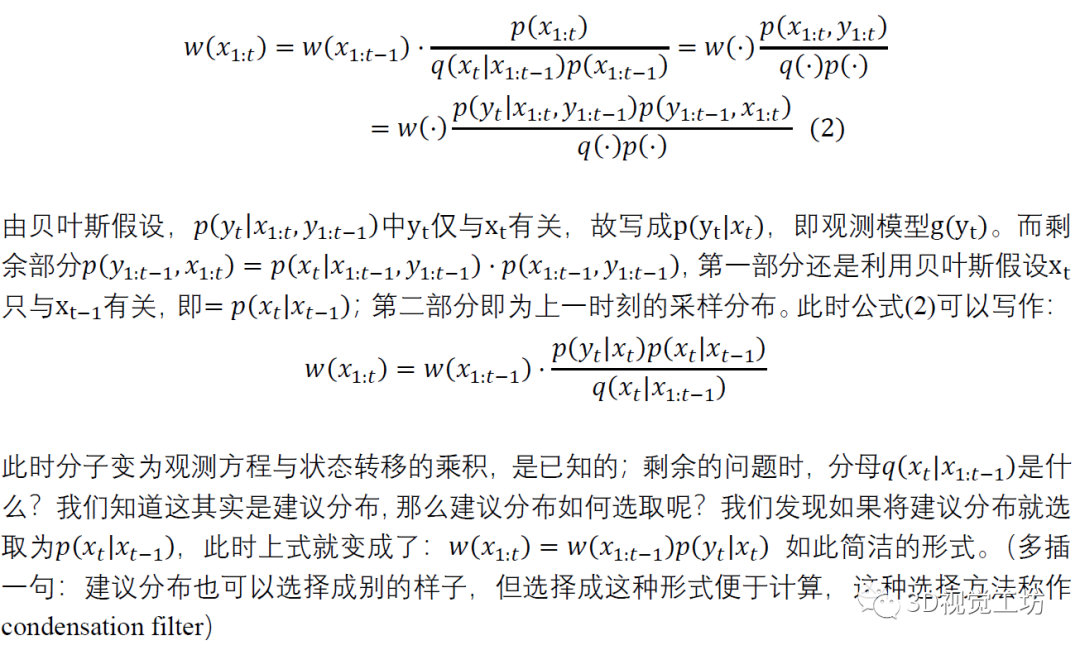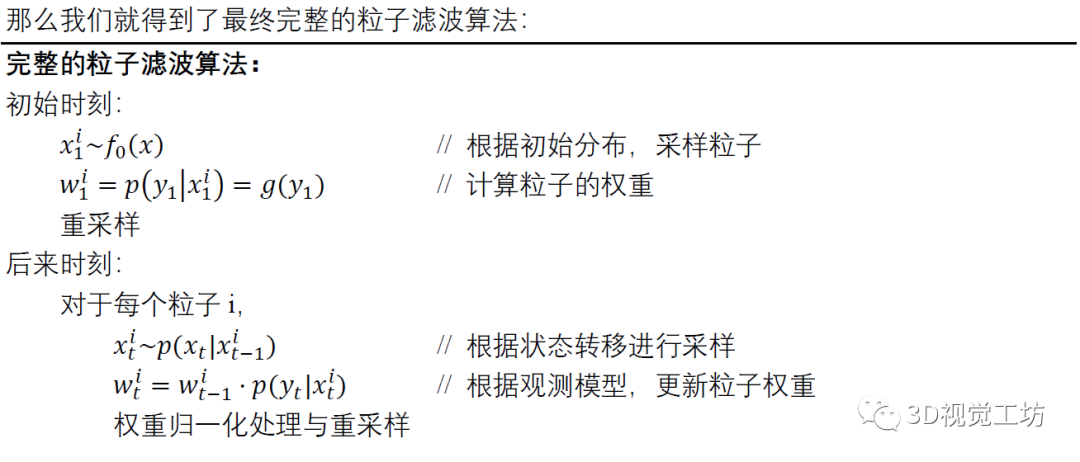# 6. 总结

本文首先从滤波问题说起，指出了贝叶斯滤波框架下积分很难求的问题。由此引出蒙特卡洛方法。之后为了降低误差、减少运算量和避免权重集中，对应出现了重要性采样、序列重要性采样与重采样，顺理成章的得出了粒子滤波的数学原理，之后给出了对应的物理模型。最后给出了简单的粒子滤波的完整算法。

作者希望通过本文，能够使得大家对粒子滤波的学习有一个完整的认识，知道粒子滤波之前有什么，而不是上来就对着资料直接学习粒子滤波本身。网络上的学习资料甚多，在这里只推荐一个：徐亦达机器学习Particle Filter：https://www.bilibili.com/video/BV1xW411N7f1?p=1。耐心看完，会有收获。

备注：作者也是我们「3D视觉从入门到精通」特邀嘉宾：一个超干货的3D视觉学习社区

本文仅做学术分享，如有侵权，请联系删文。

下载1

在「计算机视觉工坊」公众号后台回复：深度学习，即可下载深度学习算法、3D深度学习、深度学习框架、目标检测、GAN等相关内容近30本pdf书籍。

下载2

在「计算机视觉工坊」公众号后台回复：计算机视觉，即可下载计算机视觉相关17本pdf书籍，包含计算机视觉算法、Python视觉实战、Opencv3.0学习等。

下载3

在「计算机视觉工坊」公众号后台回复：SLAM，即可下载独家SLAM相关视频课程，包含视觉SLAM、激光SLAM精品课程。

##圈里有高质量教程资料、可答疑解惑、助你高效解决问题觉得有用，麻烦给个赞和在看~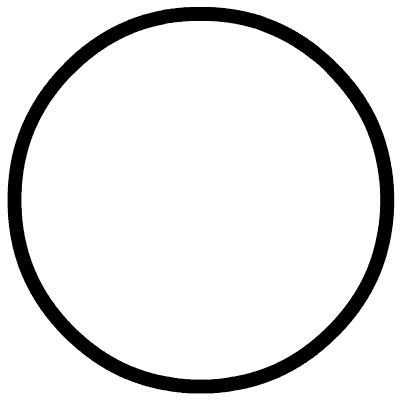展开全文算法 机器学习 人工智能 计算机视觉 编程语言
• 崔岩的笔记——粒子滤波原理及应用（3）粒子滤波原理及算法流程 粒子滤波是基于蒙特卡洛仿真的近似贝叶斯滤波算法。 我们可以从贝叶斯滤波的过程来相应的给出粒子滤波的过程。算法 目标跟踪 机器学习
• 粒子滤波通过 非参数化的蒙特卡洛 (Monte Carlo) 模拟方法来实现递推贝叶斯滤波 ，适用 于任何能用状态空间模型描述的非线性系统， 精度可以逼近最优估计。 粒子滤波器具有简单、 易于实现等特点， 它为分析非线性...
• 我们知道，科尔曼滤波限制噪声时服从高斯分布的，但是粒子滤波可以不局限于高斯噪声，原理上粒子滤波可以驾驭所有的非线性、非高斯系统。 一个比喻： 某年月，警方（跟踪程序）要在某个城市的茫茫人海（采样空间）中...算法
• ## 粒子滤波

千次阅读 2019-11-27 09:51:00
2. 粒子滤波 2.1 概念 2.2 粒子滤波算法原理 2.3 局限性 3. 代码实现 1. 状态空间模型 状态空间模型是动态时域模型，以隐含着的时间为自变量。状态空间模型在经济时间序列分析中的应用正在迅速增加。其中...
• 粒子滤波就是指通过寻找一组在状态空间中传播的随机样本来 近似的表示概率密度函数用样本均值代替积分运算进而获得系统 状态的最小方差估计的过程这些样本被形象的称为粒子故而叫 粒子滤波粒子滤波通过非参数化的...
• 粒子滤波算法原理及Matlab程序(专题).ppt》由会员分享，可在线阅读，更多相关《粒子滤波算法原理及Matlab程序(专题).ppt(18页珍藏版)》请在人人文库网上搜索。1、粒子滤波算法原理及Matlab程序,主讲： 方牛娃 QQ: ...
• 粒子滤波就是指通过寻找一组在状态空间中传播的随机样本来近似的表示概率密度函数用样本均值代替积分运算进而获得系统状态的最小方差估计的过程这些样本被形象的称为粒子故而叫粒子滤波粒子滤波通过非参数化的...
• 粒子滤波算法matlab程序，文件格式为txt格式，方便复制粘贴使用，每条语句都有注释，非常适合粒子滤波方法初学者
• 粒子滤波算法作为一种基于贝叶斯估计的非线性滤波算法，在处理非高斯非线性时变系统的参数估计和状态滤波问题方面有独到的优势。文中以弹目相对运动方程作为系统的状态方程，目标的机动模型采用Singer模型，以导引头...
• 鉴于卡尔曼滤波（Kalman Filter，KF）和粒子滤波（Particle Filter，PF）都是贝叶斯估计的一种，粒子滤波比卡尔曼滤波应用广泛，而卡尔曼滤波比粒子滤波使用简便，提出了一种算法在卡尔曼滤波和粒子滤波之间切换的...
• 查来查去，发觉粒子滤波算法（又叫MC算法）应该算是最流行的了。因此开始学习使用之。入手的是本英文书叫“probalistic robotic” 很不错，我所见到的讲得最好的一本书。花了大量时间去研读。在这里我想谈谈我对粒子...
• ## 粒子滤波定位

千次阅读 2020-03-29 21:10:07
除了线性状态估计的KF和非线性状态估计的EKF，还有一种可以解决非线性、非高斯问题的粒子滤波算法，粒子滤波主要基于蒙特卡洛方法，使用粒子集来表示概率。 粒子滤波主要分为四部分：初始化、预测、粒子权重更新、重...
• 粒子滤波实现刀具寿命预测（附python代码） 背景介绍 刀具失效是加工过程中的主要问题，通过多特征融合方法实现刀具磨损量预测后建立了刀具的健康指标。接下来就是利用得到的健康指标对刀具的剩余寿命进行预测。粒子...python...